## Ex 3.7  Question 1.

Renu purchases two bags of fertilizer of weights 75 kg and 69 kg. Find the maximum value of weight which can measure the weight of the fertilizer exact number of times.
Solution:
To find the maximum value of weight which can measure the given weight exact number of time = HCF of 75 g and 69 kgThe common factor is 3.
∴ HCF of 75 and 69 = 3.
The required maximum value of weight = 3 kg

## Ex 3.7 Question 2.

Three boys.step off together from the same spot. Their steps measure 63 cm, 70 cm, and 77 cm respectively. What is the minimum distance each should cover so that all can cover the distance in complete steps?
Solution:
For finding the minimum distance, we have to find LCM of 63, 70, and 77, we use the division method.∴ LCM of 63, 70 and 77 = 2 x 3 x 3 x 5 x 7 x 11 = 6930
The required minimum distance = 6930 cm.

## Ex 3.7 Question 3.

The length, breadth, and height of a room are 825 cm, 675 cm, and 450 cm respectively. Find the longest tape which can measure the three dimensions of the room exactly.
Solution:
The measure of the longest tape = HCF of 825, 675 and 450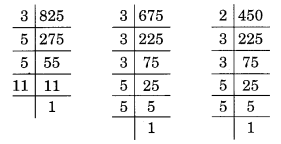825 = 3 x 5 x 5 x 11

675 = 3 x 3 x 3 x 5 x 5
450 = 2 x 3 x 3 x 5 x 5

Here, common factors are 3, 5 (two times).
∴ HCF of 825, 675 and 450 = 3 x 5 x 5 = 75
The required longest tape = 75 cm.

## Ex 3.7  Question 4.

Determine the smallest 3-digit number which is exactly divisible by 6, 8, and 12.
Solution:
The smallest 3-digit number = 100
LCM of 6, 8, and 12 –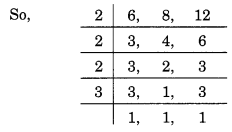∴ LCM of 6, 8 and 12 = 2 x 2 x 2 x 3 = 24
All the multiples of 24 will also be divisible by 6, 8, and 12.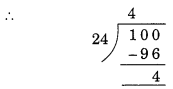100 = (100 – 4) + 24 = 96 + 24 = 120
Hence, the required number is 120.

## Ex 3.7 Question 5.

Determine the greatest 3-digit number exactly divisible by 8, 10, and 12.
Solution:
LCM of 8, 10 and 12 –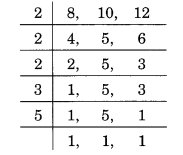∴ LCM of 8, 10 and 12 = 2 x 2 x 2 x 3 x 5 = 120
The largest 3-digit number = 999The required number i= 999 – 39 = 960.

## Ex 3.7 Question 6.

The traffic lights at three different road crossings change after every 48 seconds, 72 seconds and 108 seconds respectively. If they change simultaneously at 7 a.m., at what time will they change simultaneously again?
Solution:
LCM of 48, 72 and 108,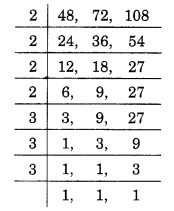∴ LCM = 2 x 2 x 2 x 2 x 3 x 3 x 3= 432
So, after 432 seconds, the light will change simultaneously.
∴The required time = 432 seconds = 7 minutes 12 seconds i.e., 7 minutes 12 seconds past 7 a.m.

## Ex 3.7 Question 7.

Three tankers contain 403 litres, 434 litres and 465 litres of diesel respectively. Find the maximum capacity of a container that can measure the diesel of the three containers the exact number of times.
Solution:
Maximum capacity of the required measure =  HCF of 403, 434 and 465.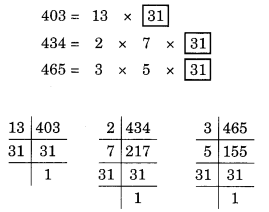Common factor = 31.
The HCF of 403, 434 and 465 = 31.
The maximum capacity of the required container = 31 litres.

## Ex 3.7 Question 8.

Find the least number which when divided by 6, 15 and 18 leave remainder 5 in each case.
Solution:
LCM of 6, 15 and 18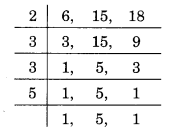∴ LCM of 6, 15 and 18 = 2 x 3 x 3 x 5 = 90.
The required number = 90 + 5 = 95.

## Ex 3.7 Question 9.

Find the smallest 4-digit number which is divisible by 18, 24 and 32.
Solution:
The smallest 4-digit number = 1000.
LCM of 18, 24 and 32∴ LCM = 2 x 2 x 2 x 2 x 2 x 3 x 3 = 288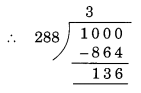So, the multiple of 288 just above 1000 = 1000 +(136 – 288) = 1152.
The required number is 1152.

## Ex 3.7 Question 10.

Find the LCM of the following numbers:
(a) 9 and 4
(b) 12 and 5
(c) 6 and 5
(d) 15 and 4
Observe a common property in the obtained ’ LCMs. Is LCM the product of two numbers in each case?
Solution:

(a) 9 and 4∴ LCM = 2 x 2 x 3 x 3 = 36.

(b) 12 and 5∴ LCM = 2 x 2 x 3 x 5 = 60.

(c) 6 and 5∴ LCM = 2 x 3 x 5 = 30.

(d) 15 and 4∴ LCM = 2 x 2 x 3 x 5 = 60.

## Ex 3.7 Question 11.

Find the LCM of the following numbers in which one number is the factor of the other.
(a) 5, 20
(b) 6, 18
(c) 12, 48
(d) 9, 45
What do you observe in the results obtained?
Solution:
(a) 5 and 20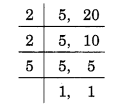∴ LCM = 2 x 2 x 5 = 20.

(b) 6 and 18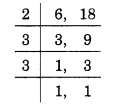∴ LCM = 2 x 3 x 3 = 18.

(c) 12 and 48LCM = 2 x 2 x 2 x 2 x 3 = 48.

(d) 9 and 45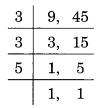∴ LCM = 3 x 3 x 5 = 45.
Hence, the LCM of 9 and 45 = 45.

error: Content is protected !!